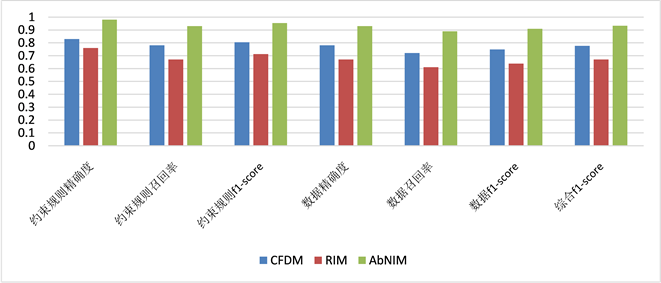﻿ 基于反常项集的异常值处理算法

# 基于反常项集的异常值处理算法An Anomalies Processing Algorithm Based on Abnormal Itemsets

Abstract: Anomalies refer to the noise and inconsistent values in the data. The detection and processing of anomalies often depend on domain constraints, which usually include conditional functional de-pendencies, negative constraints and editing rules, etc. However, for specific domains, these domain constraint rules need to be made by domain experts, and it is difficult to find these domain con-straint rules efficiently based on data mining and machine learning algorithms. In this paper, a concept of abnormal itemset for data cleaning is proposed. Similar to the outlier detection algo-rithm based on data distribution density, abnormal itemset is an unlikely combination of abnormal values in data. Then, some characteristics of lifting degree are introduced to mine abnormal itemset with low lifting degree by using the improved equivalence class transformation algorithm. Fur-thermore, this paper proposes an anomalies repair algorithm similar to the nearest neighbor in-terpolation algorithm to ensure the repair quality. Experiments under the real estate information data set show that the anomalies detection and processing algorithm based on abnormal itemset have high accuracy and will not introduce new anomalies by data repairing.

1. 引言

2. 相关工作

3. 加权调和提升度及其性质

3.1. 基于信息熵的属性权重

$H\left({A}_{k}\right)=\text{AEntropy}\left({A}_{k}\right)=-\underset{i=1}{\overset{n}{\sum }}\text{ }\text{ }P\left({a}_{i}\right){\mathrm{log}}_{2}P\left({a}_{i}\right)$ (1)

${\omega }_{k}=\frac{\text{AEntropy}\left({A}_{k}\right)-{\text{AEntropy}}_{\mathrm{min}}}{{\text{AEntropy}}_{\mathrm{max}}-{\text{AEntropy}}_{\mathrm{min}}}+1$ (2)

$H\left(M\right)=H\left({A}_{1},{A}_{2},\cdots ,{A}_{n}\right)=-\underset{x\in {A}_{1}}{\sum }\underset{x\in {A}_{2}}{\sum }\cdots \underset{x\in {A}_{n}}{\sum }\text{ }\text{ }P\left({a}_{1},{a}_{2},\cdots ,{a}_{n}\right){\mathrm{log}}_{2}P\left({a}_{1},{a}_{2},\cdots ,{a}_{n}\right)$ (3)

$\mathrm{max}\left[H\left({A}_{1}\right),H\left({A}_{2}\right),\cdots ,H\left({A}_{n}\right)\right]\le H\left({A}_{1},{A}_{2},\cdots ,{A}_{n}\right)\le H\left({A}_{1}\right)+H\left({A}_{2}\right)+\cdots +H\left({A}_{n}\right)$ (4)

$H{\left(M\right)}^{*}=H{\left({A}_{1},{A}_{2},\cdots ,{A}_{n}\right)}^{*}=\mathrm{max}\left[H\left({A}_{1}\right),H\left({A}_{2}\right),\cdots ,H\left({A}_{n}\right)\right]+\sqrt[n]{{\prod }_{i=1}^{n}H\left({A}_{i}\right)}$ (5)

3.2. 加权项集相关概念

$\text{lift}\left(A,B\right)=\frac{P\left(A,B\right)}{P\left(A\right)P\left(B\right)}=\frac{P\left(B|A\right)}{P\left(B\right)}=\frac{\text{conf}\left(A⇒B\right)}{\mathrm{sup}\left(B\right)}$ (6)

$\begin{array}{c}\text{wHlift}\left(M,D\right)=\underset{\Phi \subset N\subset M}{\mathrm{max}}\left\{\frac{\text{wsup}\left(M,D\right)}{\sqrt{\text{wsup}\left(N,D\right)\ast \text{wsup}\left(M\N,D\right)}}\right\}\\ =\frac{\text{wSupCnt}\left(M,D\right)}{\underset{\Phi \subset N\subset M}{\mathrm{min}}\left\{\sqrt{\text{wSupCnt}\left(N,D\right)\ast \text{wSupCnt}\left(M\N,D\right)}\right\}}\end{array}$ (7)

3.3. 加权调和提升度的性质

${\text{wSupCnt}}_{M}^{\mathrm{max}}=\mathrm{max}\left\{\text{wSupCnt}\left(\left\{i\right\},D\right)\right\},\left(i\in L,M\subseteq L\right)$

L为反常项集时，可以用 ${\text{wSupCnt}}_{M}^{\mathrm{max}}$ 来确定L的子集加权支持度计数的下界。由于反常项集是在数据集中真实存在的，任意一个反常项集的加权支持度计数都符合 $\text{wSupCnt}\left(L,D\right)\ge 1$ 。对于反常项集L

$\text{wHlift}\left(M,D\right)\ge \frac{\text{wSupCnt}\left(M,D\right)}{\underset{\Phi \subset P\subset M}{\mathrm{min}}\left\{\sqrt{\text{wSupCnt}\left(P,D\right)\ast \text{wSupCnt}\left(N\P,D\right)}\right\}}$

4. 反常项集挖掘算法

Eclat算法是由J. Zaki在2000年提出的，与传统的Apriori和FP-growth算法不同，Eclat (Equivalence Class Transformation，等价类变换)算法以概念格  理论为基础，利用倒排索引的思想进行数据统计，将数据转换为垂直数据表示方式，在等价类划分的思想下执行深度优先搜索来挖掘频繁项集。在垂直数据表示下，由于TID集中保存了计算支持度的完整信息，仅需对数据集进行一次数据扫描得出数据项的事务编号，再对其进行求交集操作，通过交集中的元素个数即可得出项集的支持度。倒排索引是一种更适合于做关系运算的数据结构，Eclat算法将记录划分到每个项之下，使得利用集合的简单运算就能获得所需的支持度。

1) 在Eclat算法中集成了加权调和提升度计算。在深度优先搜索概念格空间时，采取了逆序遍历的方式，使得项集M的所有子集先于项集M被遍历到，并利用额外的前缀树来存储支持度。而且，若项集M的支持度没有存储于前缀树中，意味着项集M的子集被剪枝掉了，项集M的子集不是反常项集，那么项集M和它的所有超集也不是反常项集。从而将项集M所在的分枝剪枝掉。

2) 将3.3节中加权调和提升度的性质应用于Eclat算法中，以进行剪枝和优化。

5. 异常值更正算法

5.1. 准反常项集

$\text{wHlift}\left(M,D\right)=\text{wSupCnt}\left(M,D\right)/\underset{\Phi \subset N\subset M}{\mathrm{min}}\sqrt{\text{wSupCnt}\left(M,D\right)\ast \text{wSupCnt}\left(M\N,D\right)}$

$\text{wSupCnt}\left(N,D\right)\le \text{wSupCnt}\left(M\N,D\right)$ ，其中 $N\subset M$ 。则经1次数据更正后，

$\begin{array}{l}\text{mpwHlift}\left(M,N,1\right)=\mathrm{min}\left\{\frac{\text{wSupCnt}\left(M,D\right)-1}{\sqrt{\text{wSupCnt}\left(N,D\right)*\left[\text{wSupCnt}\left(M/N,D\right)-1\right]}},\\ \text{\hspace{0.17em}}\text{\hspace{0.17em}}\text{\hspace{0.17em}}\text{\hspace{0.17em}}\text{\hspace{0.17em}}\text{\hspace{0.17em}}\text{\hspace{0.17em}}\text{\hspace{0.17em}}\text{\hspace{0.17em}}\text{\hspace{0.17em}}\text{\hspace{0.17em}}\text{\hspace{0.17em}}\text{\hspace{0.17em}}\text{\hspace{0.17em}}\text{\hspace{0.17em}}\text{\hspace{0.17em}}\text{\hspace{0.17em}}\text{\hspace{0.17em}}\text{\hspace{0.17em}}\text{\hspace{0.17em}}\text{\hspace{0.17em}}\text{\hspace{0.17em}}\text{\hspace{0.17em}}\text{\hspace{0.17em}}\text{\hspace{0.17em}}\text{\hspace{0.17em}}\text{\hspace{0.17em}}\text{\hspace{0.17em}}\text{\hspace{0.17em}}\text{\hspace{0.17em}}\text{\hspace{0.17em}}\text{\hspace{0.17em}}\text{\hspace{0.17em}}\text{\hspace{0.17em}}\text{\hspace{0.17em}}\frac{|D|\ast \text{wSupCnt}\left(M,D\right)}{\sqrt{\left[\text{wSupCnt}\left(N,D\right)+1\right]\ast \text{wSupCnt}\left(M/N,D\right)}}\right\}\end{array}$

$\text{wSupCnt}\left(N,{D}_{\text{clean}}\right)\le \text{wSupCnt}\left(N,{D}^{*}\right)\le \text{wSupCnt}\left(N,{D}_{\text{clean}}\right)+k$

$\text{wSupCnt}\left(N,{D}_{\text{clean}}\right)\ge \frac{\text{wSupCnt}\left(M,{D}^{*}\right)}{{\left({\text{wSupCnt}}_{N,{D}^{*}}^{\mathrm{max}}\ast \lambda \right)}^{2}}-k$

$\sqrt{\text{wSupCnt}\left(N,{D}_{\text{clean}}\right)}-\sqrt{\text{wSupCnt}\left(M,{D}_{\text{clean}}\right)}\ge \sqrt{\frac{1}{\lambda }}-\sqrt{{\text{wSupCnt}}_{M}^{\mathrm{max}}}-\frac{k}{|D|}$

$\text{wHlift}\left(M,{D}^{*}\right)\ge \frac{\text{wSupCnt}\left(N,{D}^{*}\right)}{\underset{S\subset I}{\mathrm{min}}\left\{\sqrt{\text{wSupCnt}\left(S,{D}_{\text{clean}}\right)+k}\ast \sqrt{\text{wSupCnt}\left(N\S,{D}_{\text{clean}}\right)+k}\right\}}$

5.2. 准反常项集挖掘算法

k表示进行预更正的次数，其取值与算法2的性能有关。接下来，对k的取值进行讨论。

5.3. 异常值更正算法

$\text{Linsim}\left(o,{o}^{\prime }\right)=\frac{{\sum }_{A\in \mathcal{A}}S\left(o\left[A\right],{o}^{\prime }\left[A\right]\right)}{{\sum }_{A\in \mathcal{A}}\mathrm{log}\left(\text{wSup}\left(\left\{\left(A,o\left[A\right]\right)\right\},D\right)\right)+{\sum }_{A\in \mathcal{A}}\mathrm{log}\left(\text{wSup}\left(\left\{\left(A,{o}^{\prime }\left[A\right]\right)\right\},D\right)\right)}$

$S\left(o\left[A\right],{o}^{\prime }\left[A\right]\right)=\left\{\begin{array}{l}2\ast \mathrm{log}\left(\text{wSup}\left(\left\{\left(A,o\left[A\right]\right)\right\},D\right)\right),\text{\hspace{0.17em}}\text{\hspace{0.17em}}\text{\hspace{0.17em}}\text{\hspace{0.17em}}\text{\hspace{0.17em}}\text{\hspace{0.17em}}\text{\hspace{0.17em}}\text{\hspace{0.17em}}\text{\hspace{0.17em}}\text{\hspace{0.17em}}\text{\hspace{0.17em}}\text{\hspace{0.17em}}\text{\hspace{0.17em}}\text{\hspace{0.17em}}\text{\hspace{0.17em}}\text{\hspace{0.17em}}\text{\hspace{0.17em}}\text{\hspace{0.17em}}\text{\hspace{0.17em}}\text{\hspace{0.17em}}\text{\hspace{0.17em}}\text{\hspace{0.17em}}\text{\hspace{0.17em}}\text{\hspace{0.17em}}\text{\hspace{0.17em}}\text{\hspace{0.17em}}\text{\hspace{0.17em}}\text{\hspace{0.17em}}\text{\hspace{0.17em}}\text{\hspace{0.17em}}\text{\hspace{0.17em}}\text{\hspace{0.17em}}\text{\hspace{0.17em}}\text{\hspace{0.17em}}\text{\hspace{0.17em}}\text{\hspace{0.17em}}\text{\hspace{0.17em}}\text{\hspace{0.17em}}\text{\hspace{0.17em}}\text{\hspace{0.17em}}\text{\hspace{0.17em}}\text{\hspace{0.17em}}\text{\hspace{0.17em}}\text{\hspace{0.17em}}o\left[A\right]={o}^{\prime }\left[A\right]\\ 2\ast \mathrm{log}\left(\text{wSup}\left(\left\{\left(A,o\left[A\right]\right)\right\},D\right)\right)+\mathrm{log}\left(\text{wSup}\left(\left\{\left(A,{o}^{\prime }\left[A\right]\right)\right\},D\right)\right),\text{\hspace{0.17em}}\text{\hspace{0.17em}}\text{\hspace{0.17em}}其他\end{array}$

6. 实验

6.1. 实验设置

6.2. 评价指标

6.3. 实验结果Figure 1. The comparison of experimental results

7. 总结

 Hemalatha, C.S., Vaidehi, V. and Lakshmi, R. (2015) Minimal Infrequent Pattern Based Approach for Mining Outliers in Data Streams. Expert Systems with Applications, 42, 1998-2012.
https://doi.org/10.1016/j.eswa.2014.09.053

 Duncan, K. and Wells, D. (1999) A Rule Based Data Cleansing. Journal of Data Warehousing, 4, 146-159.

 Szathmary, L., Napoli, A. and Valtchev, P. (2007) Towards Rare Item-set Mining. 19th IEEE International Conference on Tools with Artificial Intelligence, Patras, 29-31 October 2007, 305-312.
https://doi.org/10.1109/ICTAI.2007.30

 Kolahi, S. and Lakshmanan, L. (2009) On Approximating Optimum Repairs for Functional Dependency Violations. Database Theory-ICDT, International Conference, St Peters-burg, March 2009, 53.
https://doi.org/10.1145/1514894.1514901

 Chu, X., Ilyas, I.F. and Papotti, P. (2014) Discovering Denial Con-straints. Proceedings of the Vldb Endowment, 6, 1498-1509.
https://doi.org/10.14778/2536258.2536262

 Beskales, G., Ilyas, I.F., Golab, L. and Galiullin, A. (2012) On the Relative Trust between Inconsistent Data and Inaccurate Constraints. 2013 IEEE 29th International Conference on Data Engineering (ICDE), Brisbane, 8-12 April 2013, 541-552.
https://doi.org/10.1109/ICDE.2013.6544854

 刘波, 耿寅融. 数据质量检测规则挖掘方法[J]. 模式识别与人工智能, 2012(5): 835-844.

 林印华, 张春海, 刘洁. 基于清洗规则和主数据的数据复算法实现[J]. 计算机科学, 2012, 39(11): 174-176.

 王凤梅, 胡丽霞. 一种基于近邻规则的缺失数据填补方法[J]. 计算机工程, 2012, 38(21): 53-55.

 Szathmary, L., Valtchev, P. and Napoli, A. (2010) Generating Rare Association Rules Using the Minimal Rare Item Sets Family. International Journal of Soft-ware and Informatics, 4, 219-238.

 Adda, M., Lei, W. and Yi, F. (2007) Rare Itemset Mining. International Con-ference on Machine Learning & Applications, Cincinnati, 13-15 December 2007, 73-80.
https://doi.org/10.1109/ICMLA.2007.106

 Szathmary, L., Valtchev, P. and Napoli, A. (2010) Finding Minimal Rare Itemsets and Rare Association Rules. International Conference on Knowledge Science, Engineering and Manage-ment, Belfast, 1-3 September 2010, 16-27.
https://doi.org/10.1007/978-3-642-15280-1_5

 Ouyang, W.M. and Huang, Q.H. (2013) Mining Indirect Tem-poral Sequential Patterns in Large Transaction Databases. Applied Mechanics and Materials, 385-386, 1362-1365.
https://doi.org/10.4028/www.scientific.net/AMM.385-386.1362

 Szathmary, L., Valtchev, P., Napoli, A. and Godin, R. (2009) Efficient Vertical Mining of Frequent Closures and Generators. Advances in Intelligent Data Analysis VIII, 8th International Symposium on Intelligent Data Analysis, IDA 2009, Lyon, 31 August-2 September 2009, 393-404.
https://doi.org/10.1007/978-3-642-03915-7_34

 Zaki, M.J. and Hsiao, C.J. (2002) CHARM: An Efficient Al-gorithm for Closed Itemset Mining. Proceedings of the Second SIAM International Conference on Data Mining, Arling-ton, 11-13 April 2002, 457-473.
https://doi.org/10.1137/1.9781611972726.27

 Rammelaere, J., Geerts, F. and Goethals, B. (2017) Cleaning Data with Forbidden Itemsets. IEEE International Conference on Data Engineering, San Diego, 19-22 April 2017, 897-908.
https://doi.org/10.1109/ICDE.2017.138

 Boriah, S., Chandola, V. and Kumar, V. (2008) Similarity Measures for Categorical Data: A Comparative Evaluation. Proceedings of the SIAM International Conference on Data Mining, SDM 2008, Atlanta, 24-26 April 2008, 243-254.
https://doi.org/10.1137/1.9781611972788.22

 Chiang, F. and Miller, R.J. (2008) Discovering Data Quality Rules. Proceedings of the Vldb Endowment, 1, 1166-1177.
https://doi.org/10.14778/1453856.1453980

Top